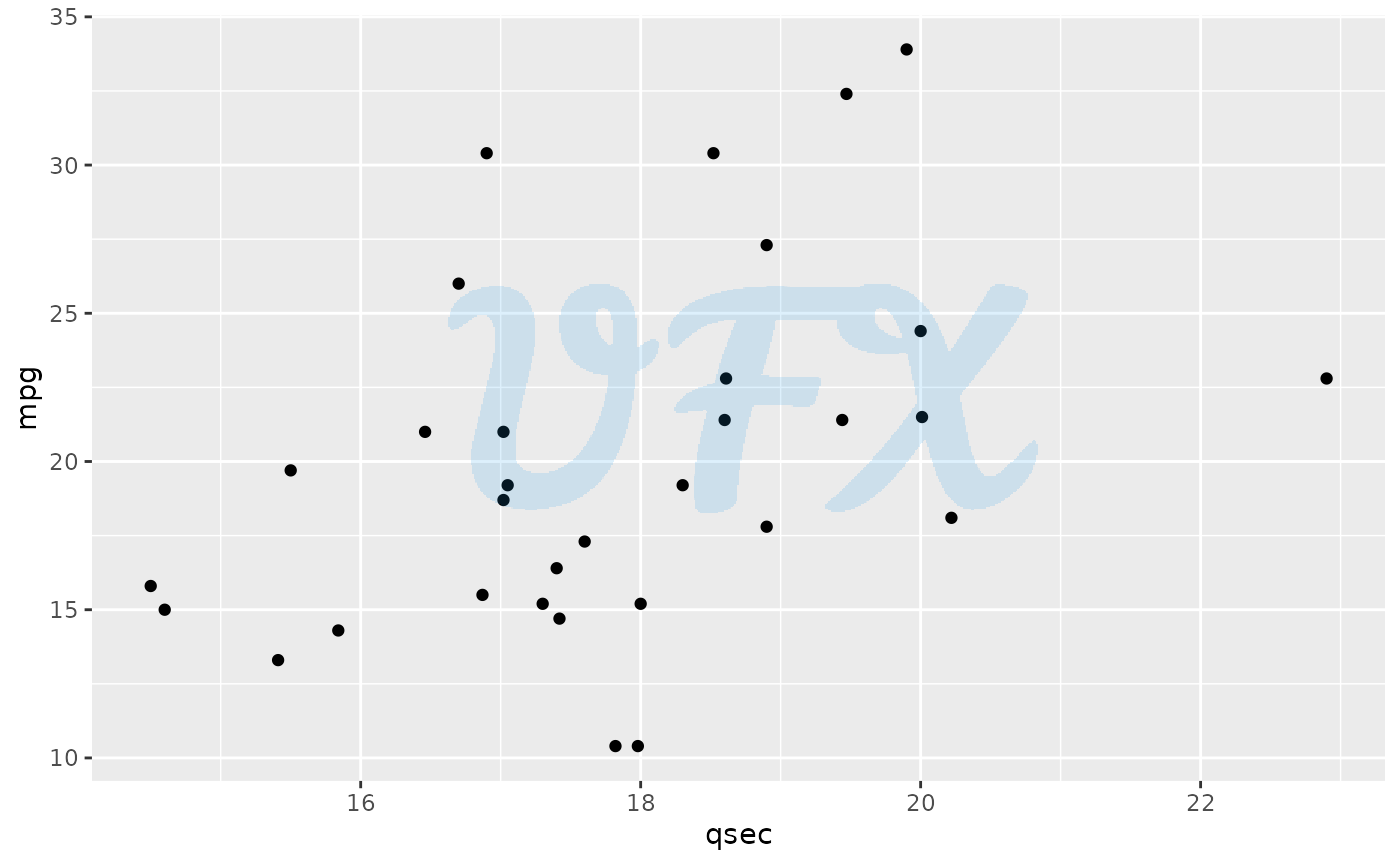library(relper)
library(ggplot2)

plt_ functions are extensions to ggplot2 objects. So let’s define a base plot to apply the functions.

plot <-
ggplot(mtcars,aes(qsec,mpg))+
geom_point()

plot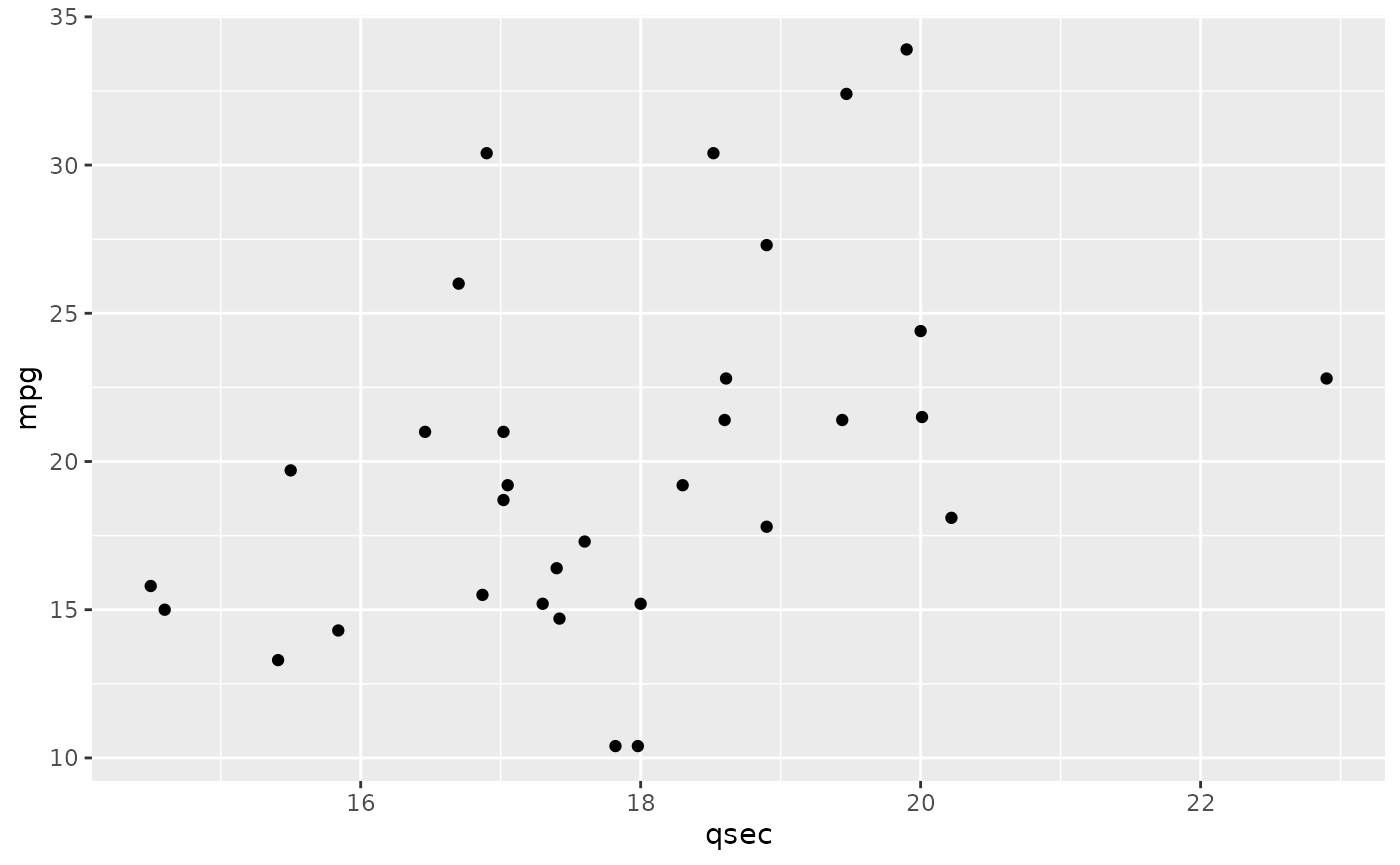## plt_flip_y_title

The goal of plt_flip_y_title is to flip the title from y axis, so it can be read horizontally.

plot + plt_flip_y_title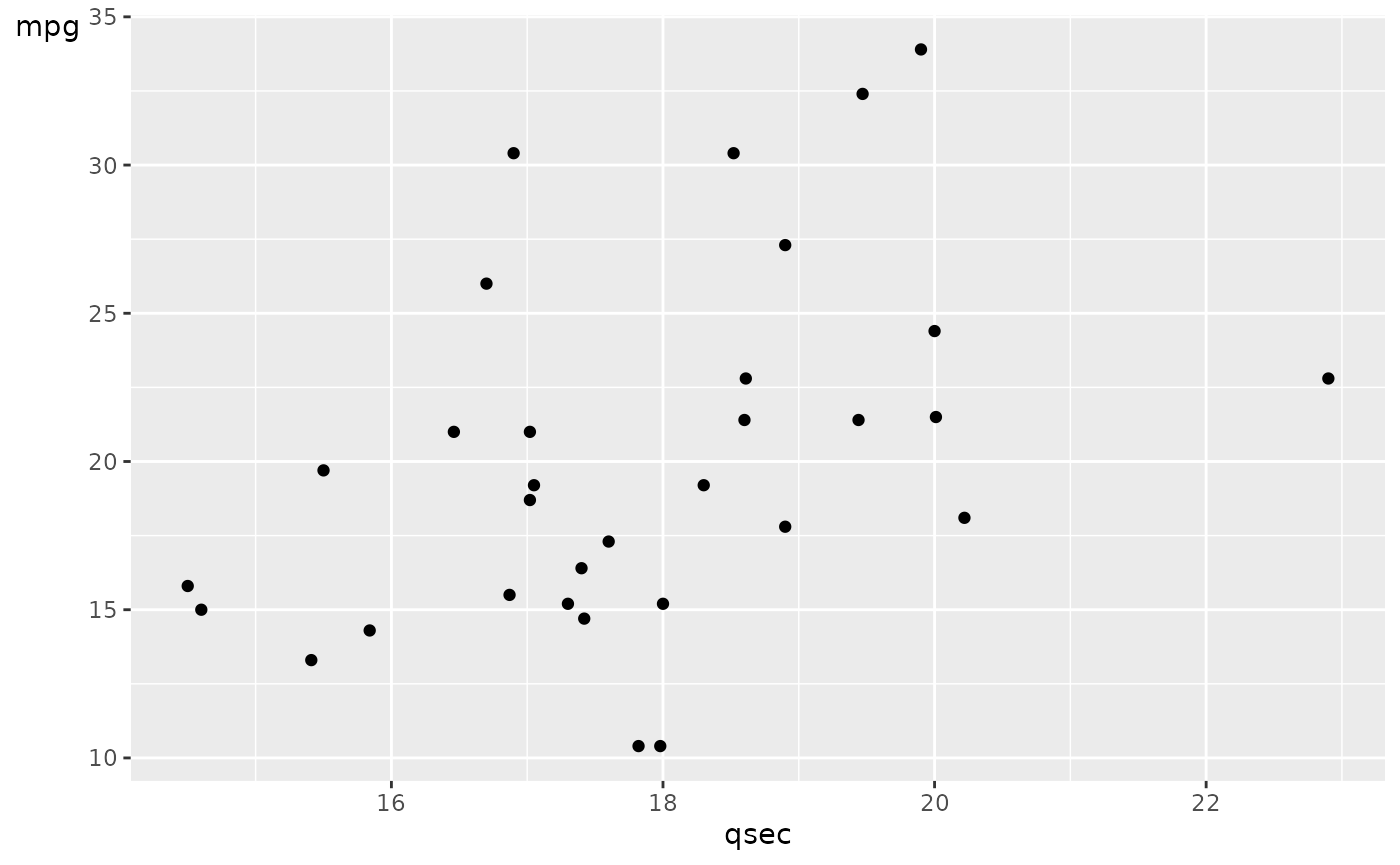## plt_identity_line

The goal of plt_identity_line is to add a identity line to a scatter plot.

plot + plt_identity_line(color = "red")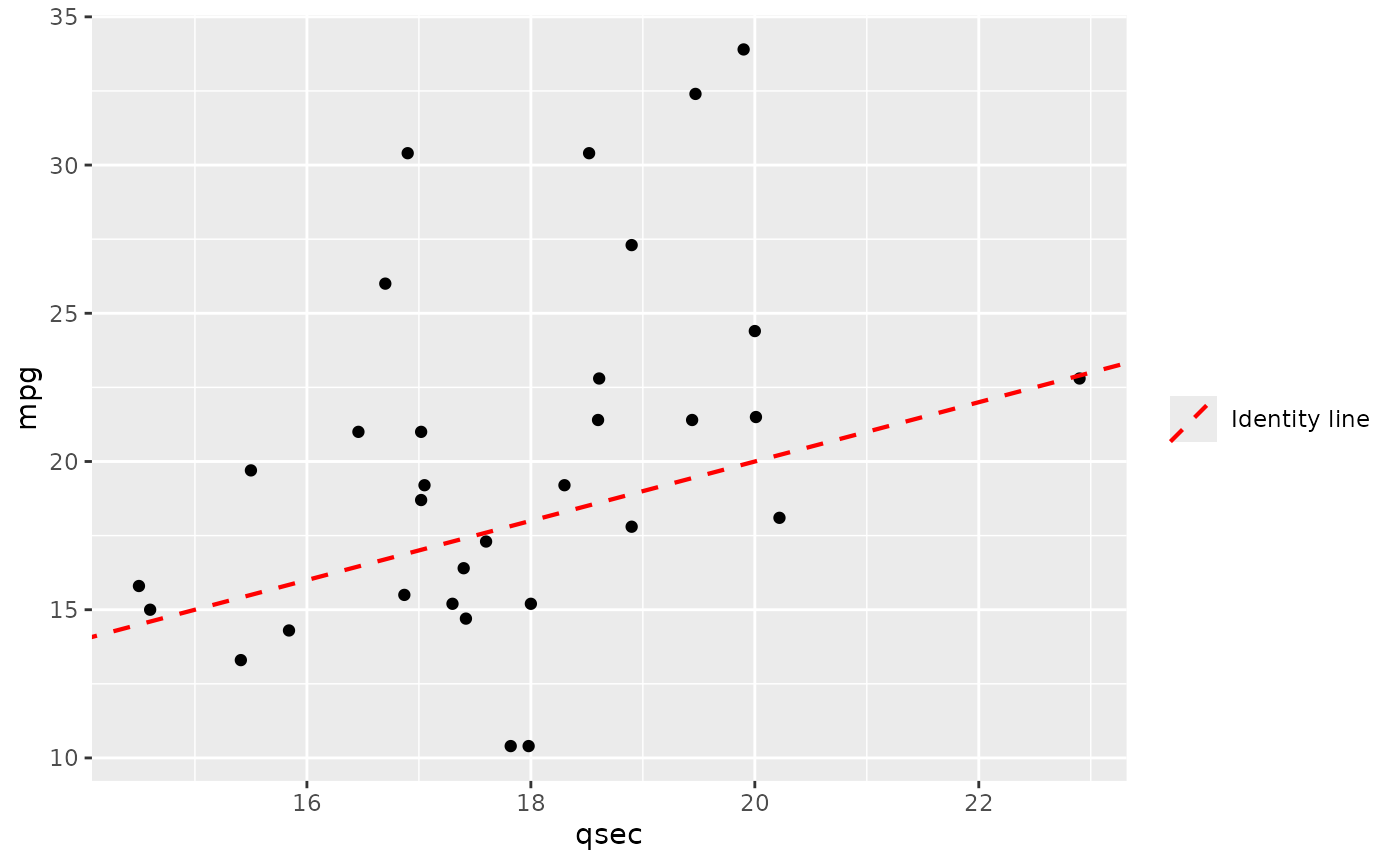## plt_no_background

The goal of plt_no_background is to remove the background.

plot + plt_no_background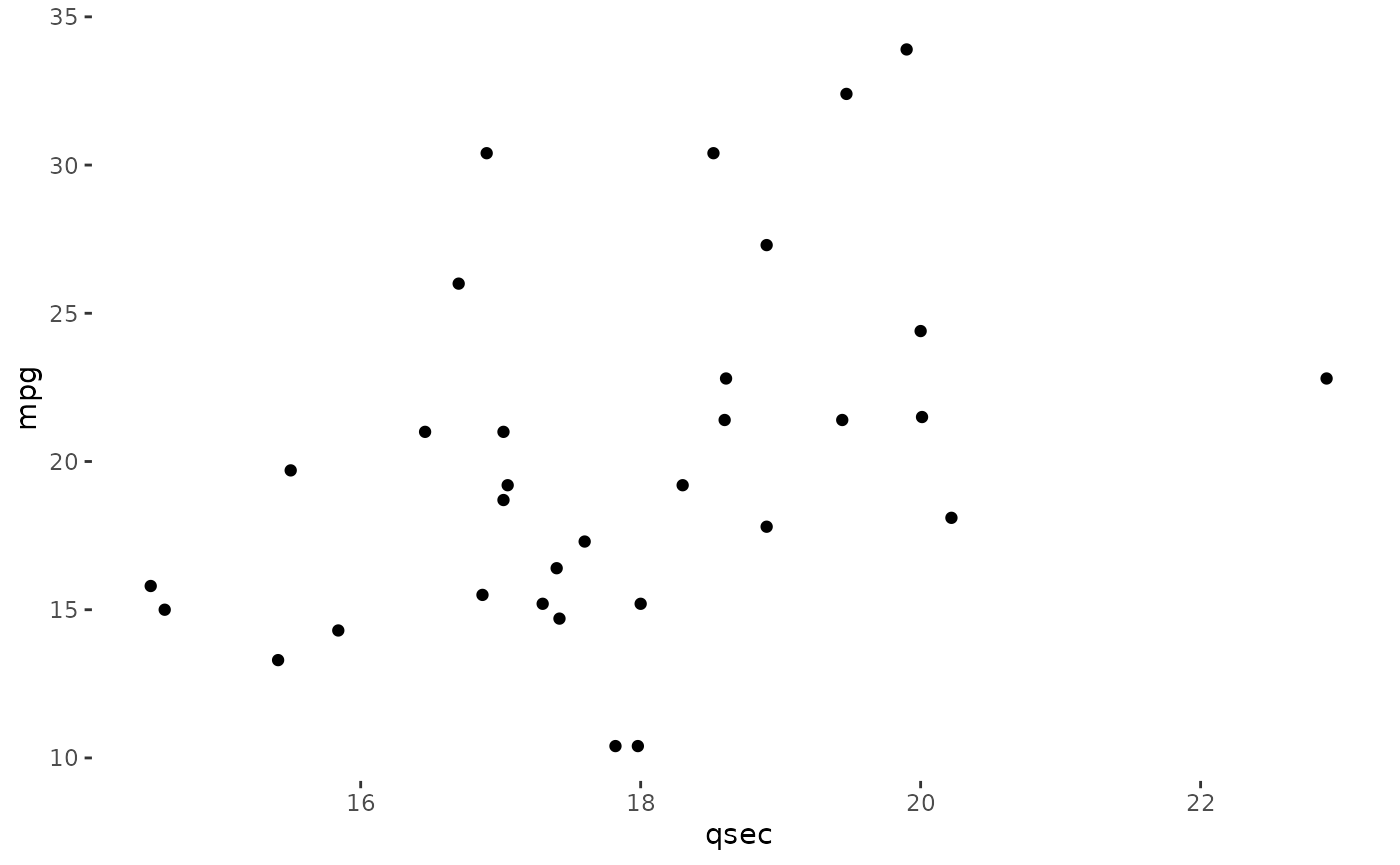## plt_no_grid

The goal of plt_no_grid is to remove the grid.

plot + plt_no_grid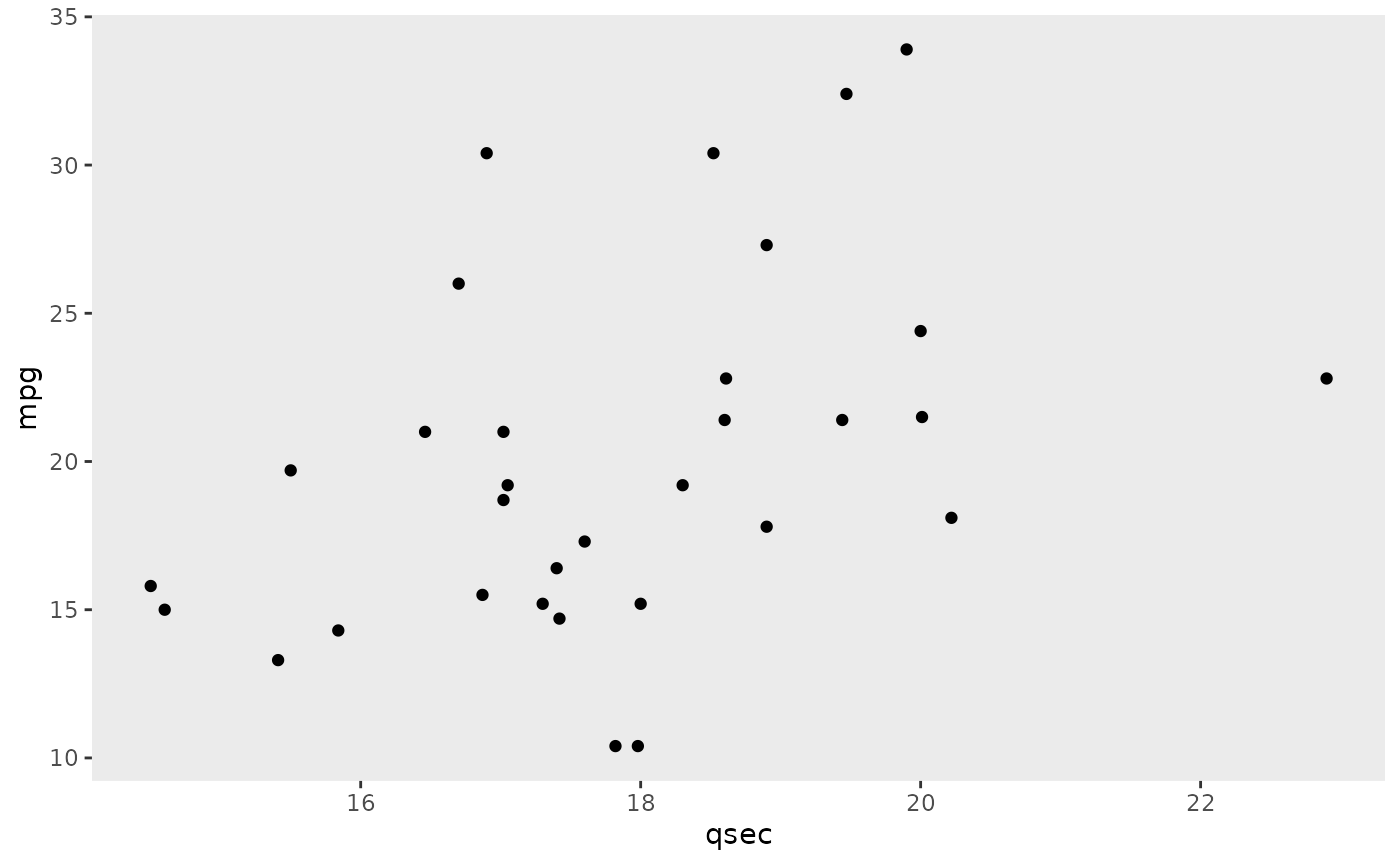## plt_no_labels

The goal of plt_no_labels is to remove all labels.

plot + plt_no_labels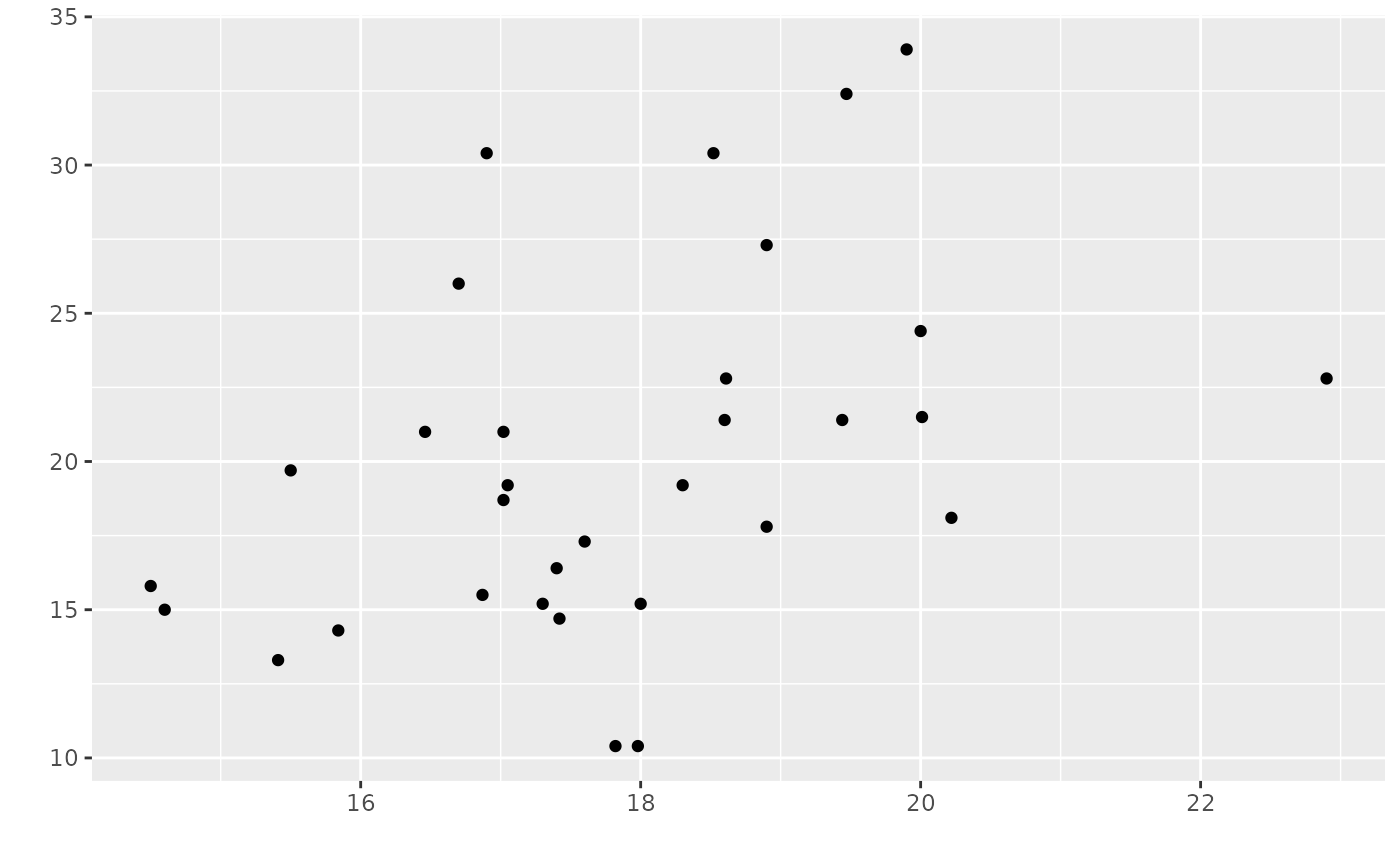## plt_no_text

The goal of plt_no_text is to remove the text and ticks.

plot + plt_no_text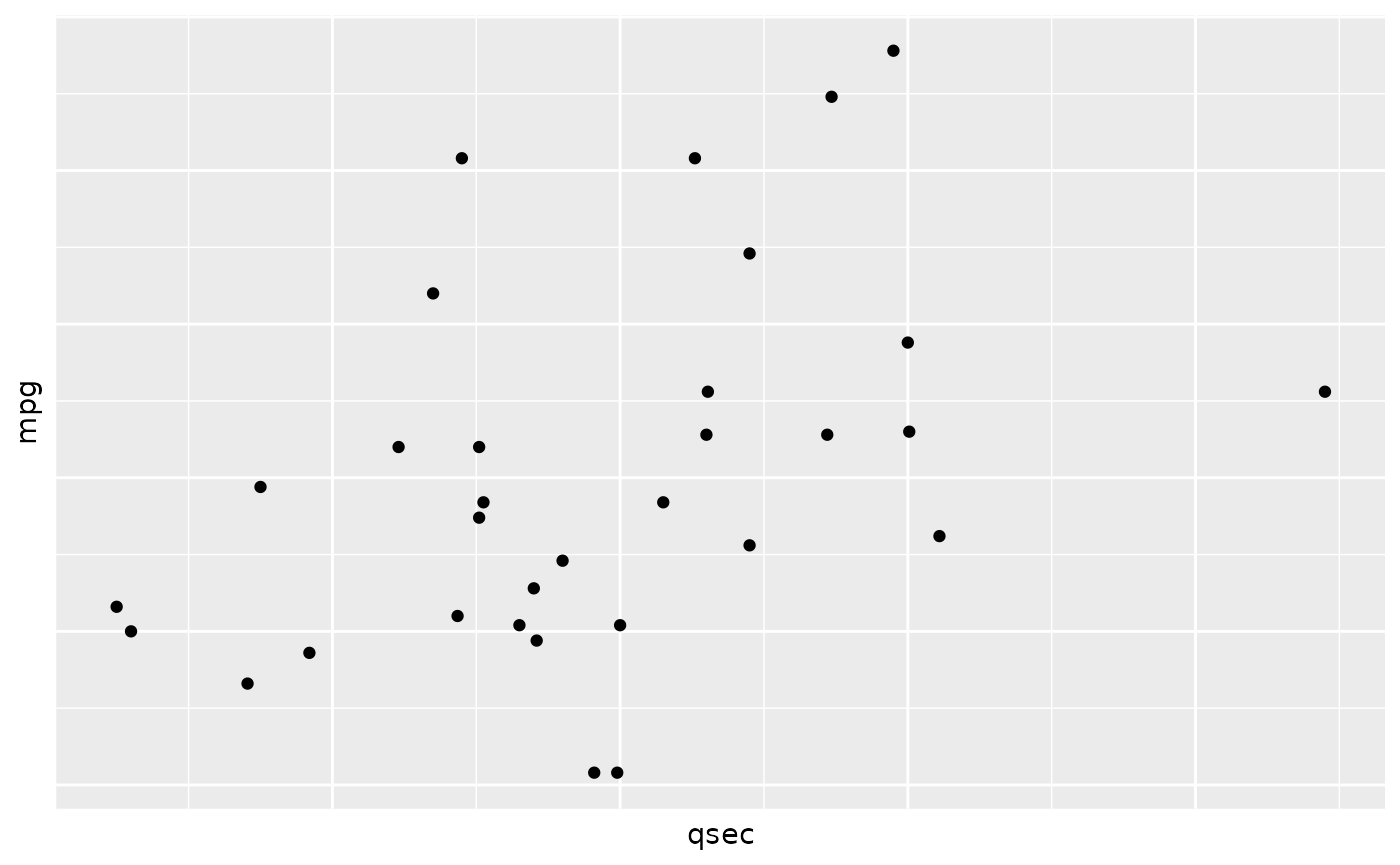## plt_pinpoint

The goal of plt_pinpoint is to add a single point to a plot.

plot + plt_pinpoint(x = 18,y = 25)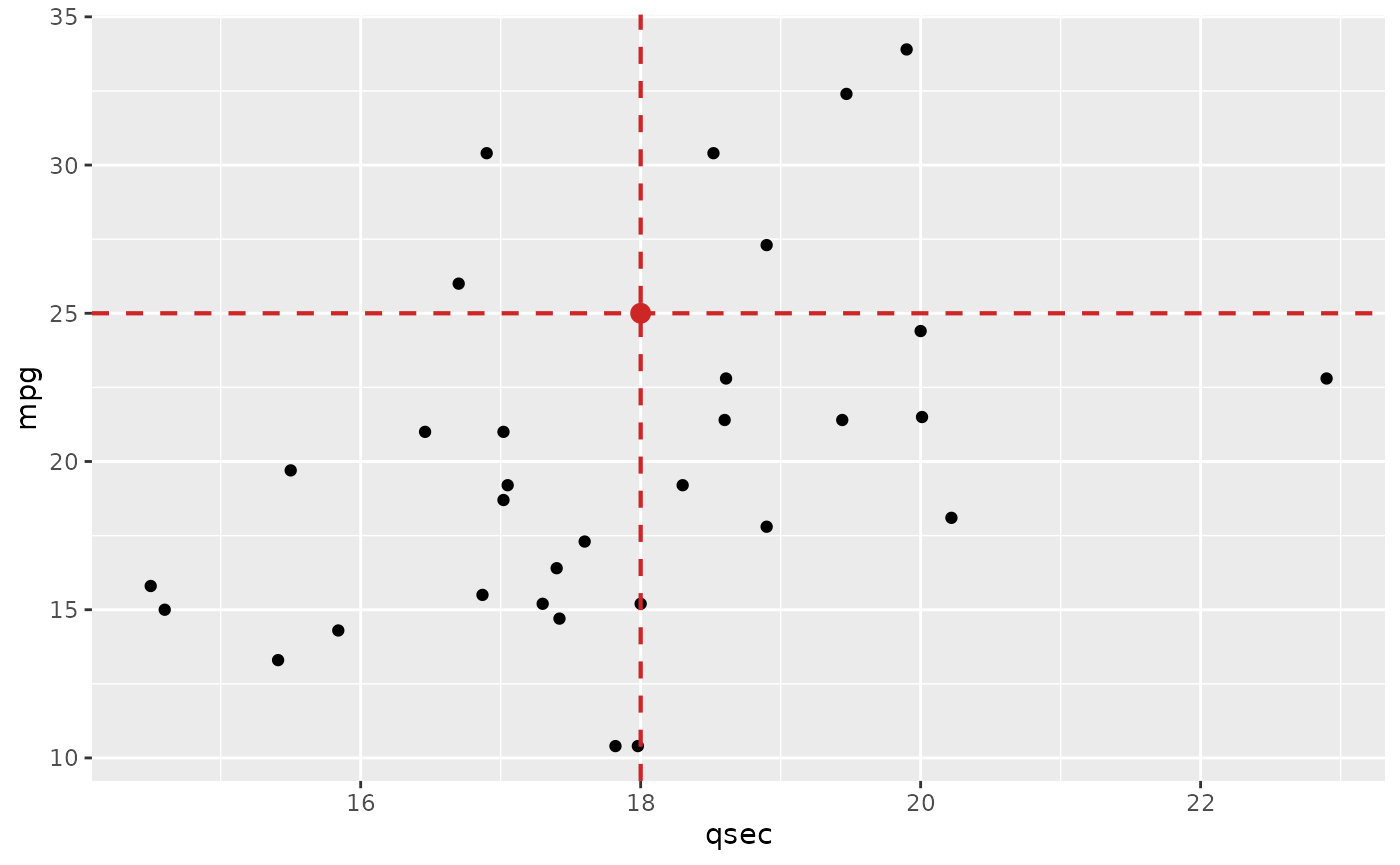## plt_regression_line

The goal of plt_regression_line is to add a regression line to a scatter plot.

plot + plt_regression_line(color = "red")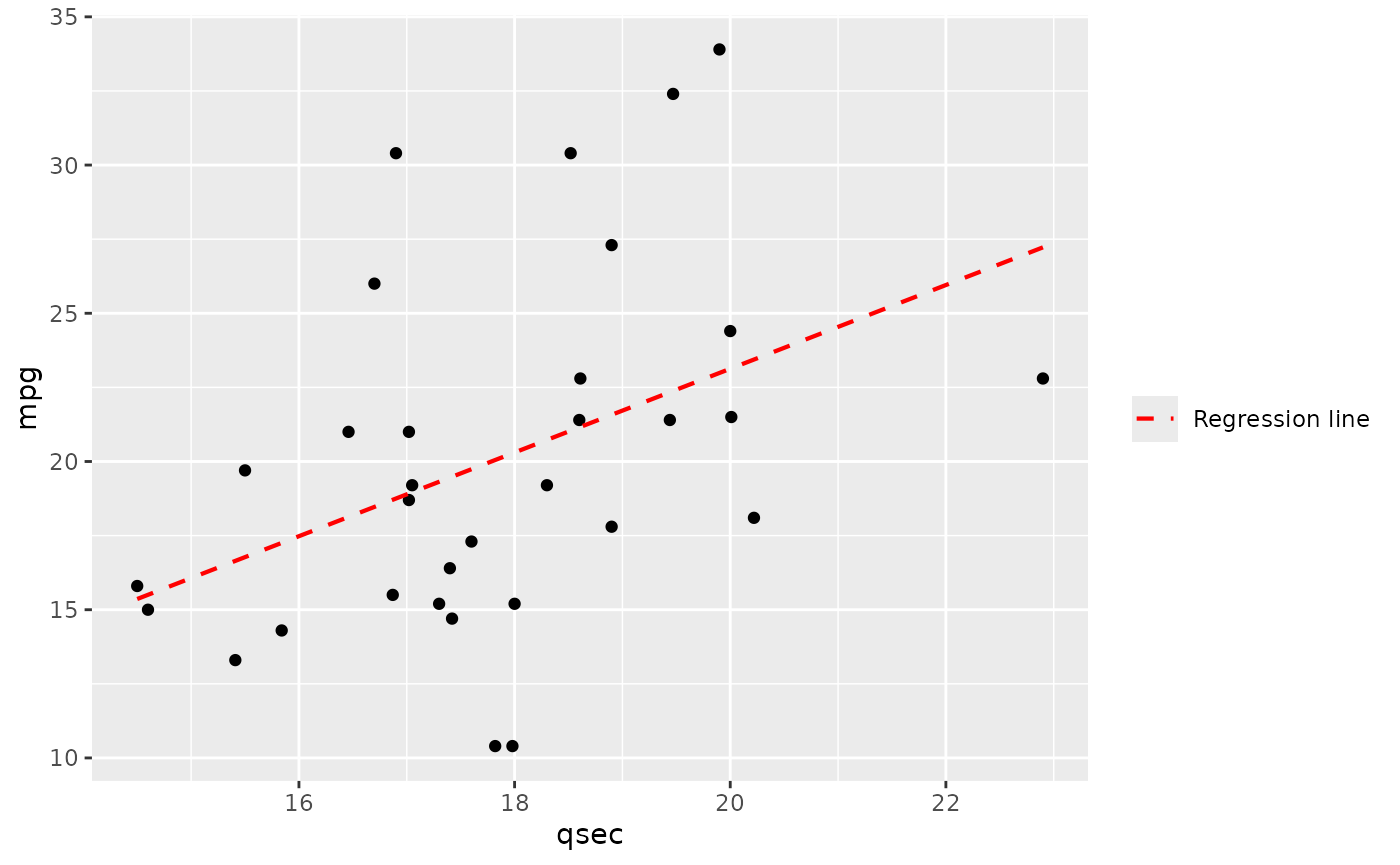## plt_scale_auto

The goal of plt_scale_auto is to add a automatic scale.

plot + plt_scale_auto(axis = "x",n_breaks = 5)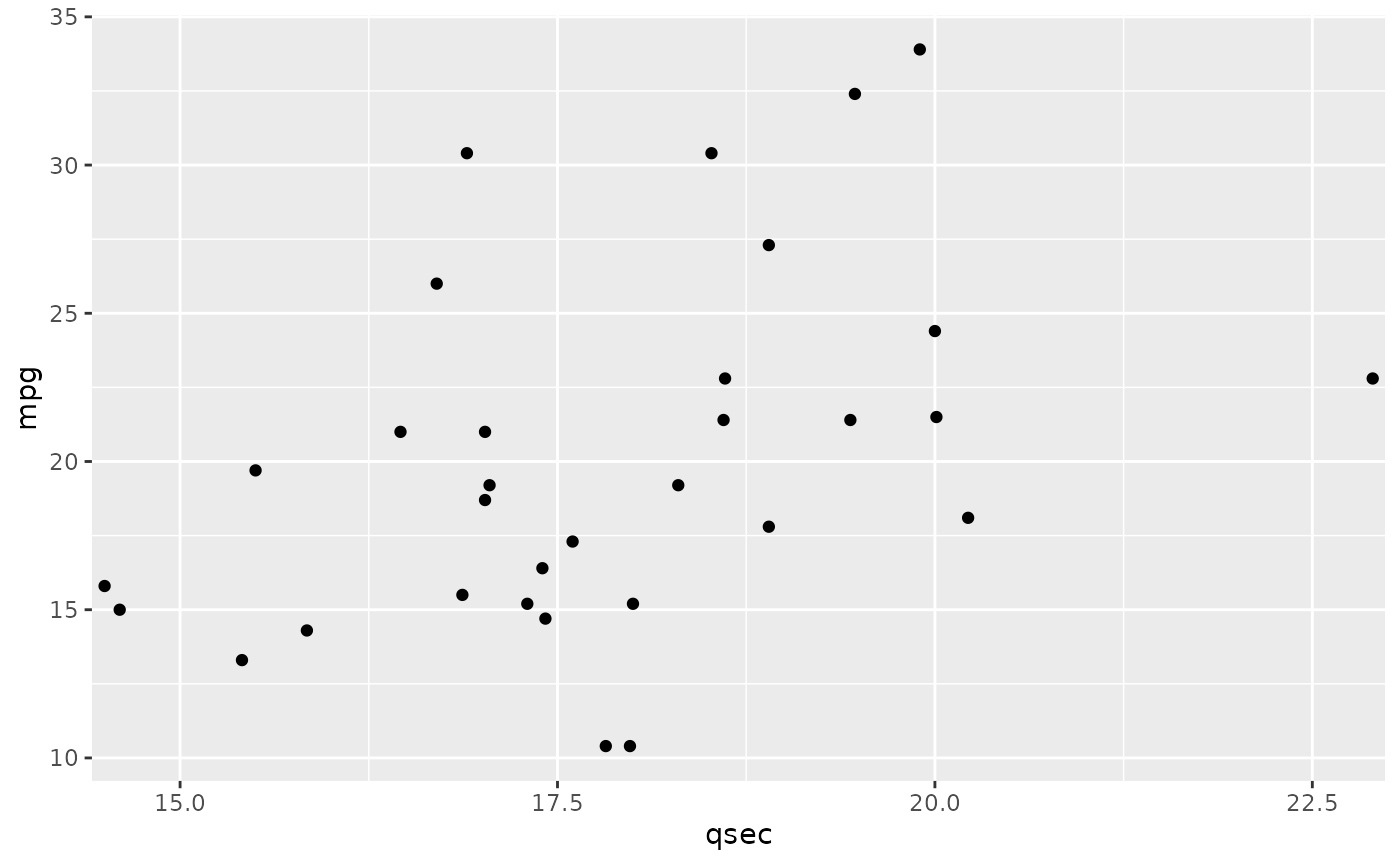plot +
plt_scale_auto(axis = "xy",n_breaks = 5)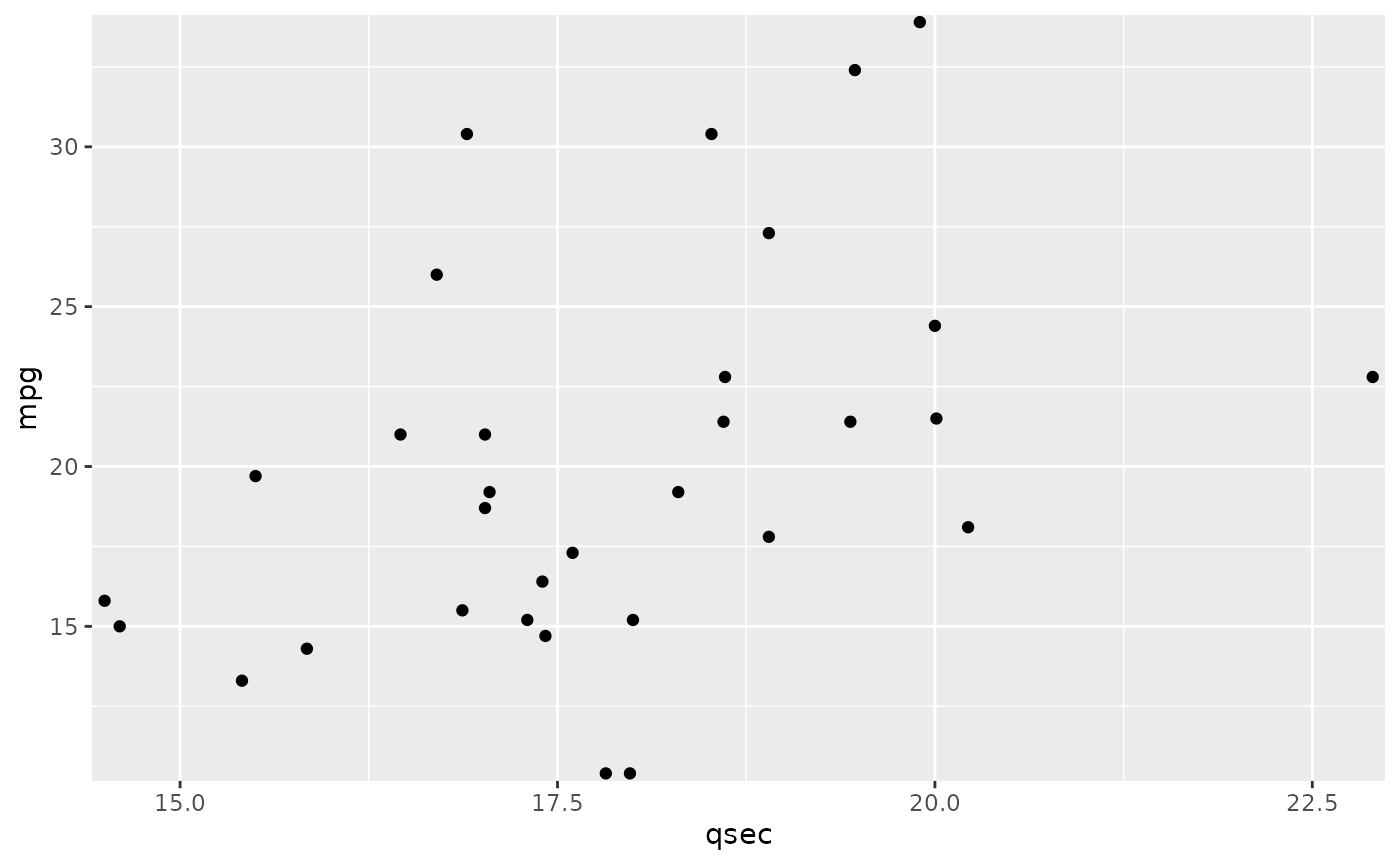## plt_scale_y_mirror

The goal of plt_scale_y_mirror is to mirror the y axis on the right side.

plot_breaks <- seq(0,35,3)

plot_labels <- format_num(plot_breaks,digits = 2)

plot + plt_scale_y_mirror(breaks = plot_breaks, labels = plot_labels)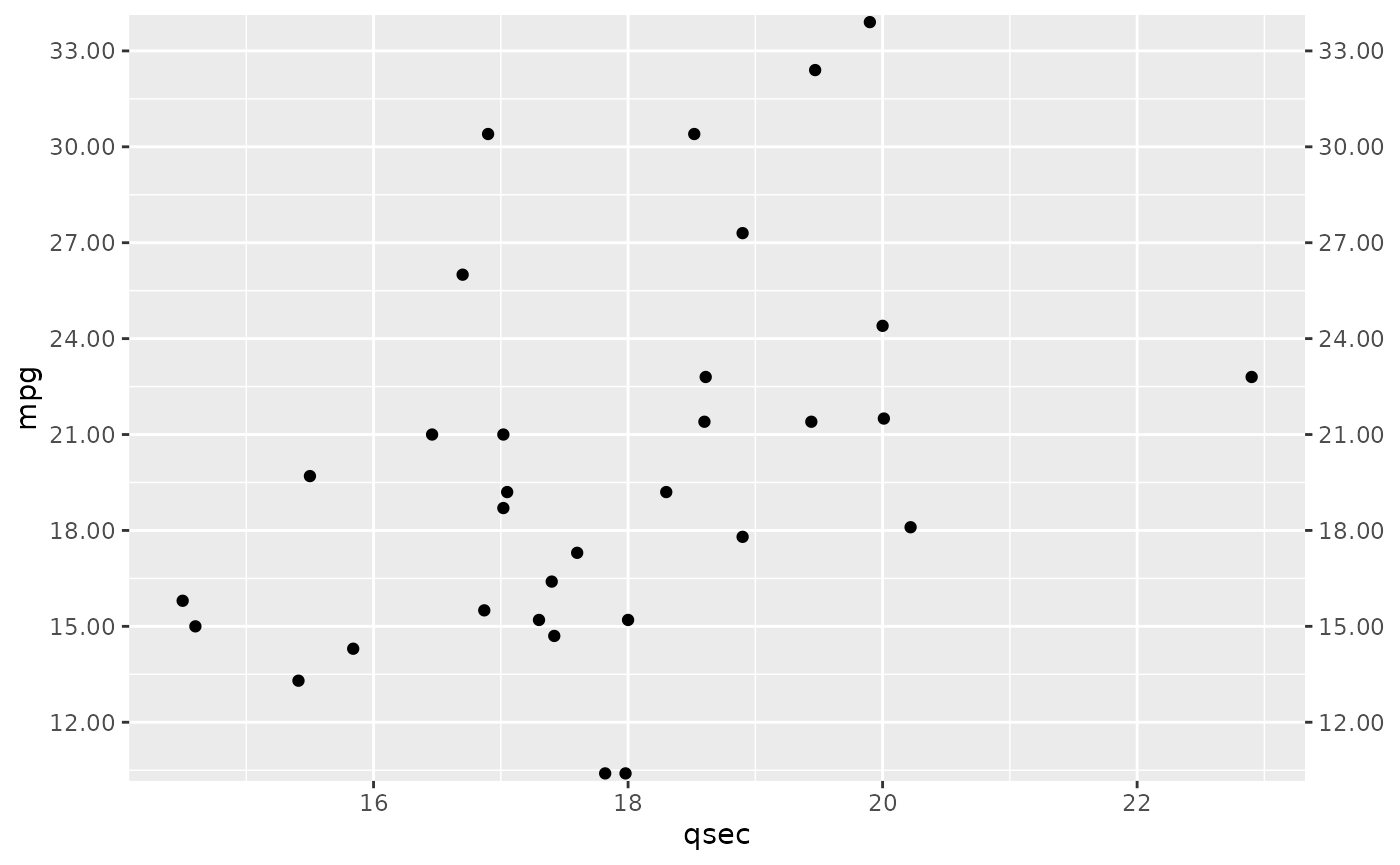## plt_theme_map

The goal of plt_theme_map is to add a theme appropriate for a map.

plot + plt_theme_map()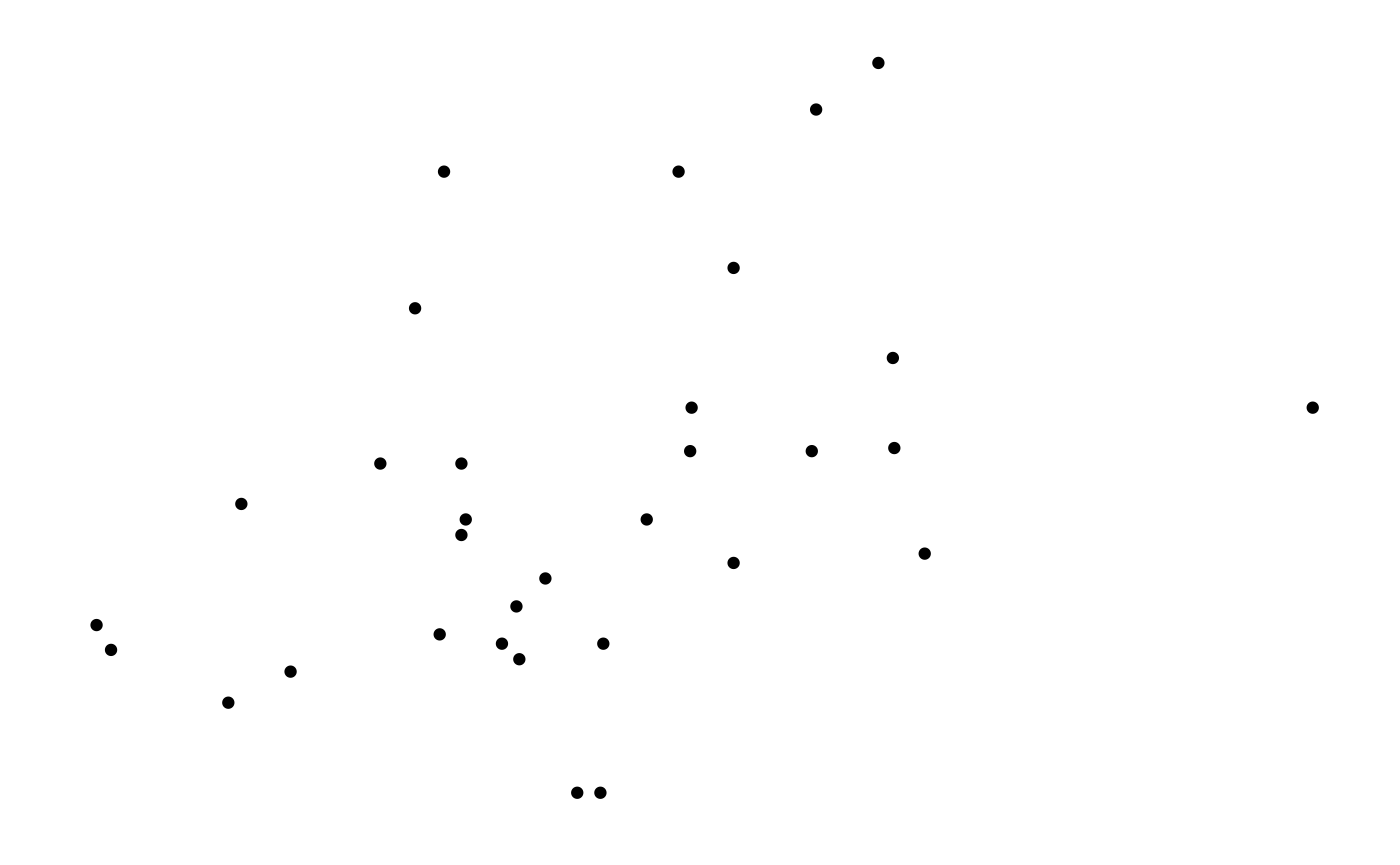## plt_theme_x

The goal of plt_theme_x is to remove major and minor grid lines from y axis.

plot + plt_theme_x(margin = 1)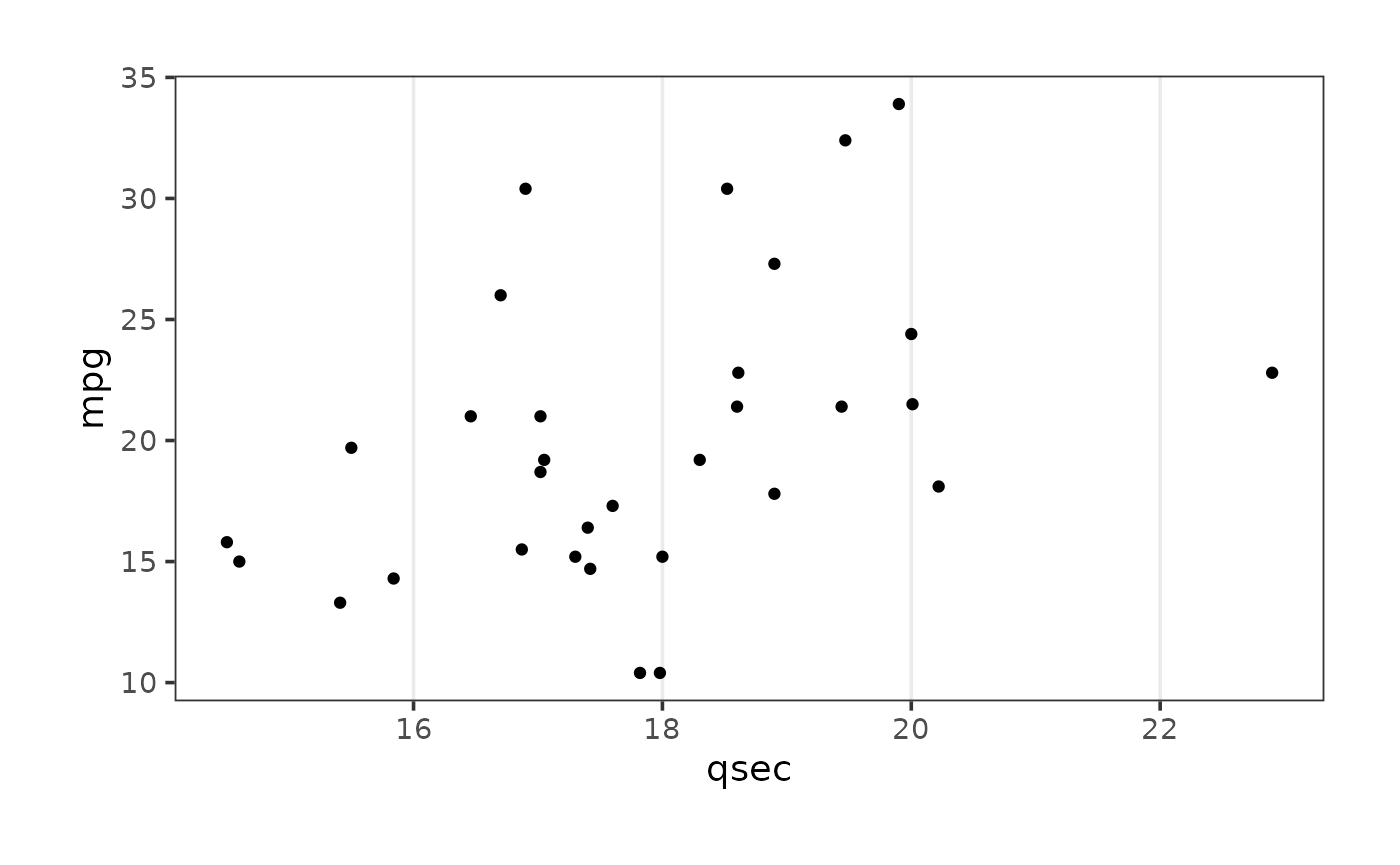## plt_theme_xy

The goal of plt_theme_xy is to remove minor grid lines from x and y axis.

plot + plt_theme_xy(margin = 2)## plt_theme_y

The goal of plt_theme_y is to remove major and minor grid lines from x axis.

plot + plt_theme_y()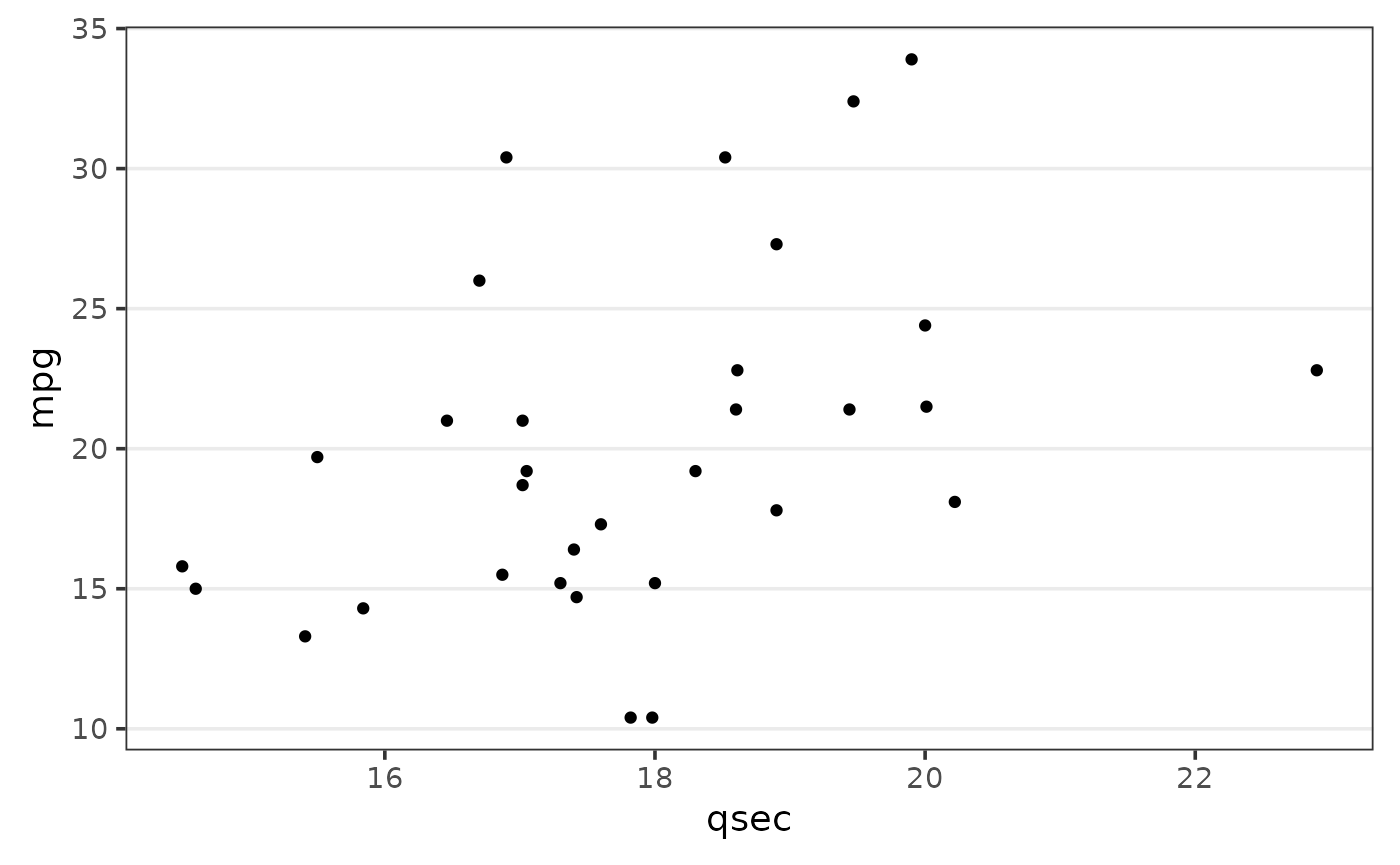## plt_water_mark

The goal of plt_water_mark is to add a image as a watermark in a ggplot2 object.

plot + plt_water_mark(vfx_watermark)